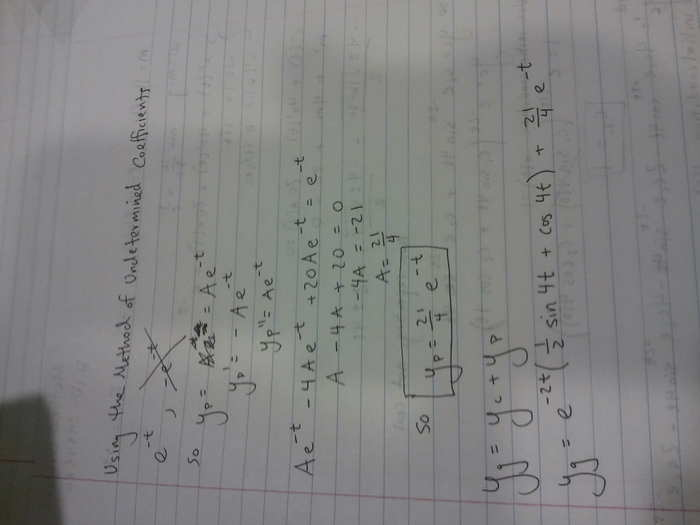# Problem with an Undamped Forced Motion

My professor assigned us a problem where
m=16 lb
k=10 lb/ft
a=2
t(0)=1 ft
t'(0)=0 ft/sec

Through this I determined the DE
x''(t)+4x'(t)+20x(t)=e-t
with initial conditions mentioned above.

Using the method of m2+4m+20=0
I got roots of -2±4i
Therefore, my yc=e-2t[c1sin(4t)+c2cos(4t)]

Next I attempted to solve further the problem, but I did not understand what to do as the examples I could find throughout the internet and my textbook all are regarding F(t)=cos(t) or similar.

Any help or guidance would be appreciated. Unfortunately the problem is due tomorrow morning so any quick help is more preferred ;)

Thanks,
Matt!

I managed to find
c1=1/2
c2=1

So
yc=e-2t((1/2)sin4t+cos4t)

Now what to doI did some more work, can someone verify that this is correct please? :)Thanks.

Also, the graph I got from my general solution is provided by this link: "[URL [Broken]

Last edited by a moderator:
I actually ended up getting y=(e^(-2x))((8/17)sin(4x)+(16/17)cos(4x))+(1/17)e^(-x)
I hope this is right :).